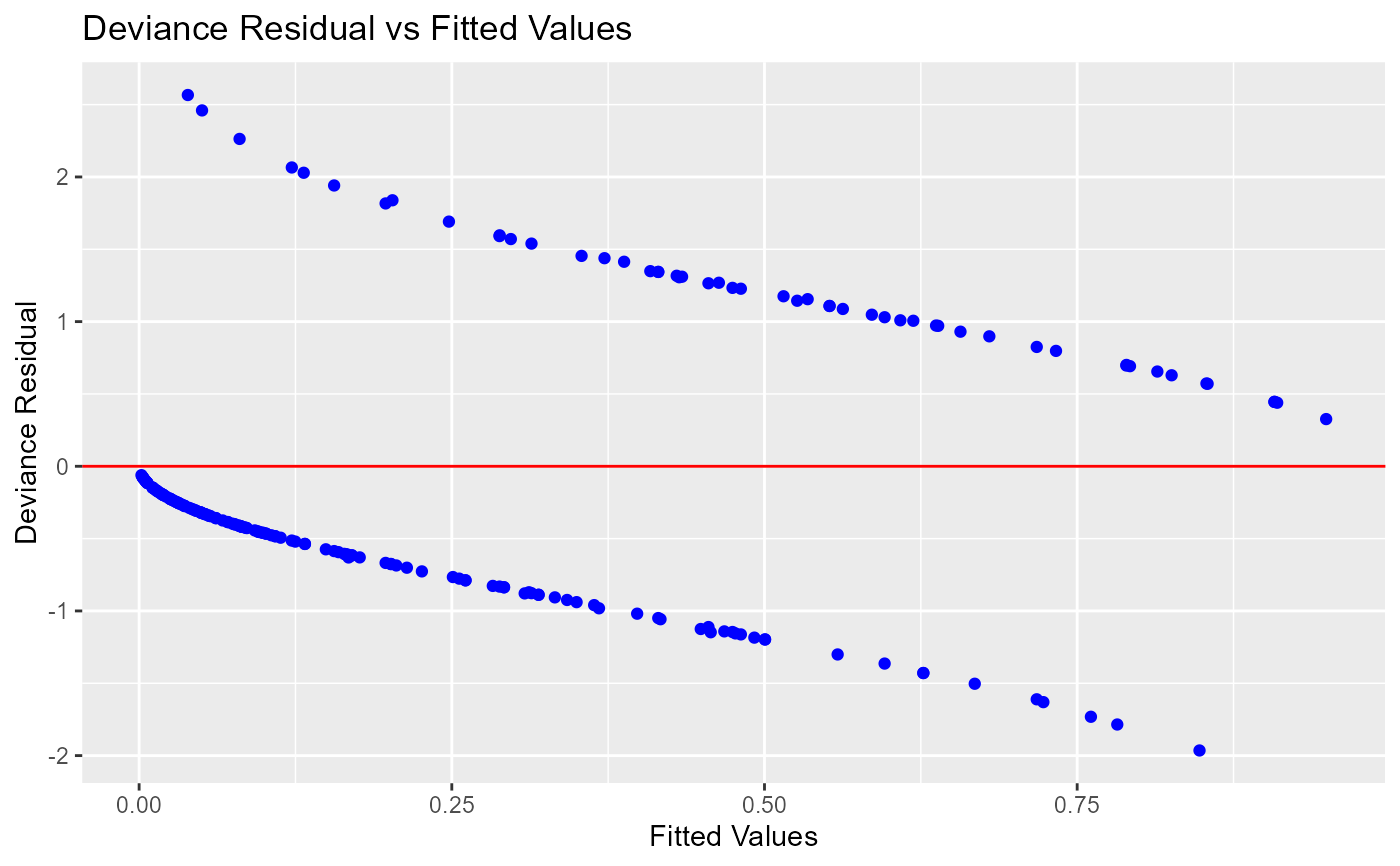Deviance vs fitted values plot.

blr_plot_deviance_fitted(
model,
point_color = "blue",
line_color = "red",
title = "Deviance Residual vs Fitted Values",
xaxis_title = "Fitted Values",
yaxis_title = "Deviance Residual"
)

## Arguments

model An object of class glm. Color of the points. Color of the horizontal line. Title of the plot. X axis label. Y axis label.

## Examples

model <- glm(honcomp ~ female + read + science, data = hsb2,
family = binomial(link = 'logit'))

blr_plot_deviance_fitted(model)